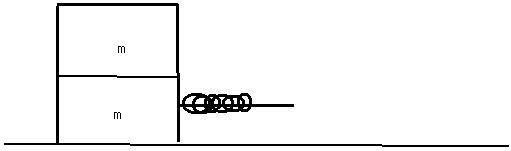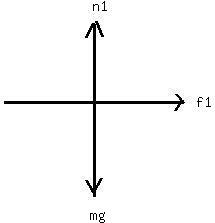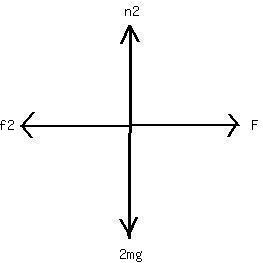Question# A 2.4-kg object is attached to a horizontal spring of forceconstant k=4.5 kN/m. The spring is stretched 10 cm fromequilibrium and released. Find (a) t

Other
ANSWEREDA 2.4-kg object is attached to a horizontal spring of forceconstant k=4.5 kN/m. The spring is stretched 10 cm fromequilibrium and released. Find (a) the frequency of themotion, (b) the period, (c) the amplitude, (d) the maximum speed,and (e) the maximum acceleration. (f) When does the objectfirst reach its equilibrium position? What is itsacceleration at this time?
Two identical blocks placed one on top of the other rest on africtionless horizontal air track. The lower block isattached to a spring of spring constant k= 600 N/m. Whendisplaced slightly from its equilibrium position, the systemoscillates with a frequency of 1.8 Hz. When the amplitude ofoscillation exceeds 5 cm, the upper block starts to slide relativeto the lower one. (a) What are the masses of the twoblocks? (b) What is the coefficient of static frictionbetween the two blocks?2021-03-26
This is very difficult to explain without free-bodydiagrams (FBD). I'll try to draw something:The stuff on the right is supposed to be a spring.
The FBD for the top mass:The bottom mass:$$\displaystyle{f}=\mu{n}=\mu{m}{g}$$
F is the force due to the spring (F=kx). Applying Newton's 2nd law to the first FBD:
$$\displaystyle\mu{m}{g}={m}{a}$$
And to the second FBD:
$$\displaystyle{k}{x}-\mu{\left({2}{m}\right)}{g}={m}{a}$$
The blocks don't slide when they have the same acceleration:
$$\displaystyle{a}=\mu{g}={\frac{{{k}{x}}}{{{m}}}}-{2}\mu{g}$$
$$\displaystyle\mu={\frac{{{k}{x}}}{{{3}{m}{g}}}}$$
m is the mass of a single block, calculated in the first partof the problem. The blocks will slide when x = 0.05; plugthis value for x into the equation and solve.